# Introduce about JShell in Java

If you have ever worked with some languages like Python, Scala, you may know we can write code and execute code for those languages using only the command line. This will allow us to test some code without having to do a lot of things, simply writing code and executing. This function is also known as REPL, Read-Eval-Print-Loop. Grabbing that essential need, from version 9, Java introduced us to a similar tool called JShell. What is it? I will have some introduction about it in this tutorial.

To start JShell, just open Terminal and enter the following statement: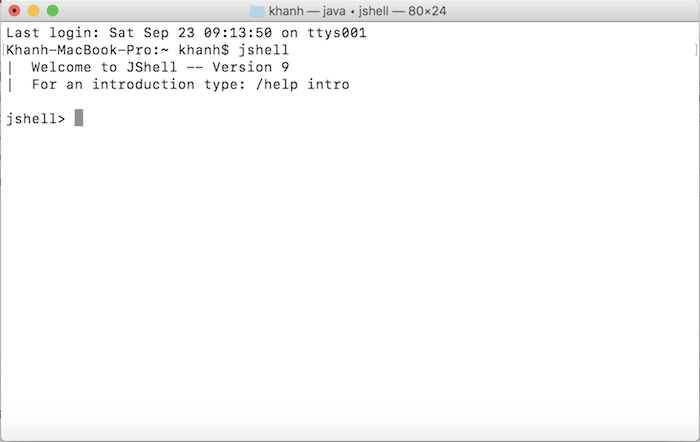Let try writing an example with System.out.print().You see, using JShell, we do not need to create new class, add main() method then run. All is simply what we want the program to do, eliminating redundant manipulation.

To find out what JShell can do for us, you can enter the command line “/ help”.Here, you will find JShell can do a lot of things for us.

For example, the import command in Java that we often use, JShell supports us by importing some packages, and so when we use it, we do not need to declare those packages with the import statement anymore. To check the packages that JShell has imported, you can enter the following statement: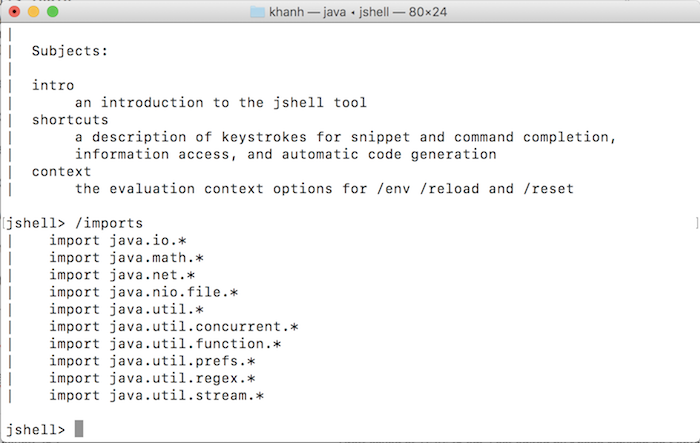For example, normally, when using the Stream object in the IDE, we will write code like this:

In JShell is simply: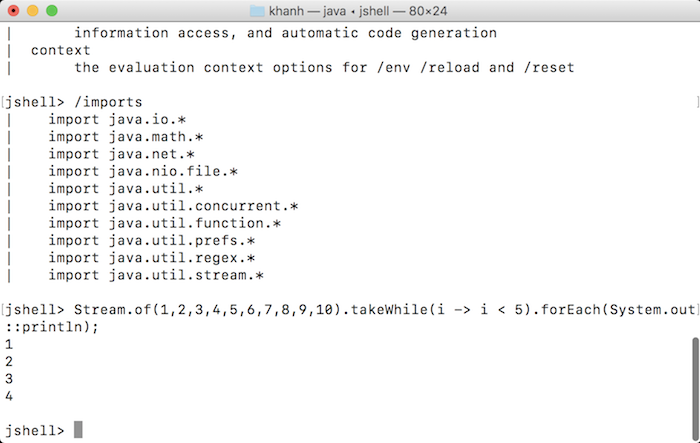JShell also has good support for methods.

For example, I define a method sum() as follows: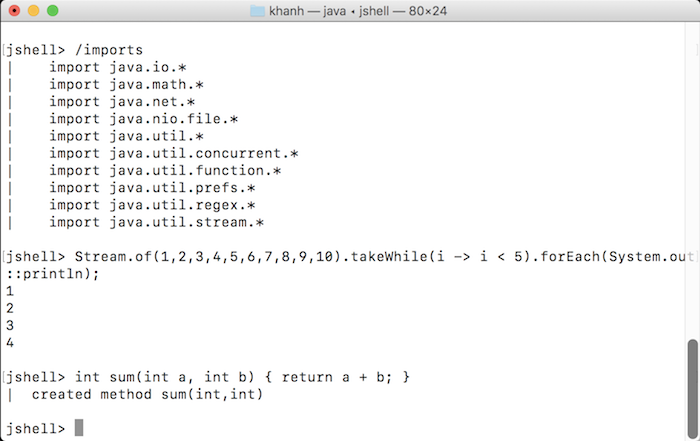Then, I can call to use the sum() method as follows:You notice that after executing the code, if the result returns a value, JShell will automatically assign that value to a variable. As an example of our sum() method, after executing the code sum(2, 3), the return value is assigned to variable \$3.

We can reuse these variables. For example, you can reprint the \$3 variable as follows: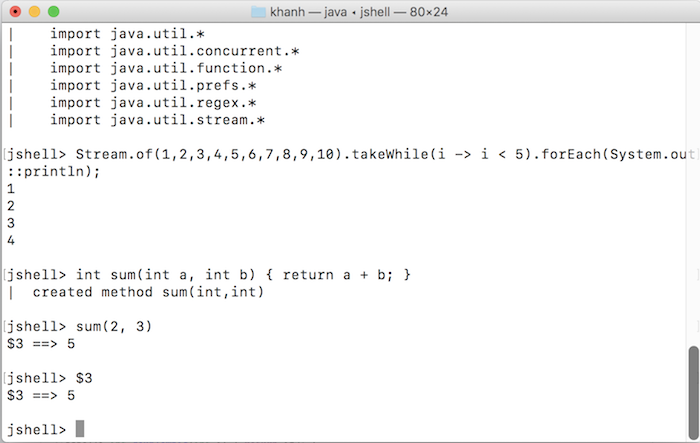To exit JShell, just enter “/exit”.